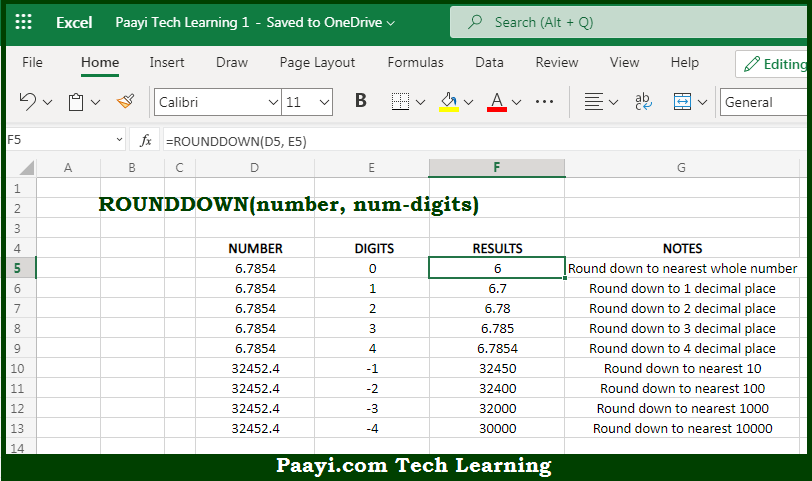# Learn How to Use Microsoft Excel ROUNDDOWN Function

Written by | 0 Comments | 533 Views

In this article, you will learn how to use the Microsoft Excel ROUNDDOWN function and its prime function in Microsoft Excel. You will also get to know the Microsoft Excel ROUNDDOWN function return value and syntax with the help of some examples.

Microsoft Excel ROUNDDOWN Function

The main function of the Microsoft Excel ROUNDDOWN function is to round a number to the given number of places. That implies, with the help of the ROUNDDOWN function you can able to return a rounded number up to a given number of places. It should be noted that the ROUNDDOWN functions is different from the ROUND function where less than five numbers are rounded down, as with ROUNDOWN function you can able to round down all numbers. So, with the help of the ROUNDDOWN function, you can able to round a number to the given number of places.

Return Value of ROUNDDOWN Function

The return value will be the rounded number.

Syntax of ROUNDDOWN Function

=ROUNDDOWN(number, num-digits)

Where the arguments:

• number: This is the number which you want to round.
• num-digits: This is the number of digits the number should be rounded.

## How to Use Microsoft Excel ROUNDDOWN Function?So we know that Microsoft Excel ROUNDDOWN function you can able to round a number to the given number of places. That implies, with the help of the ROUNDDOWN function you can able to return a rounded number up to a given number of places. It should be noted that the ROUNDDOWN functions is different from the ROUND function where less than five numbers are rounded down, as with ROUNDOWN function you can able to round down all numbers.

So, with the help of the ROUNDDOWN function, you can able to round a number to the given number of places.